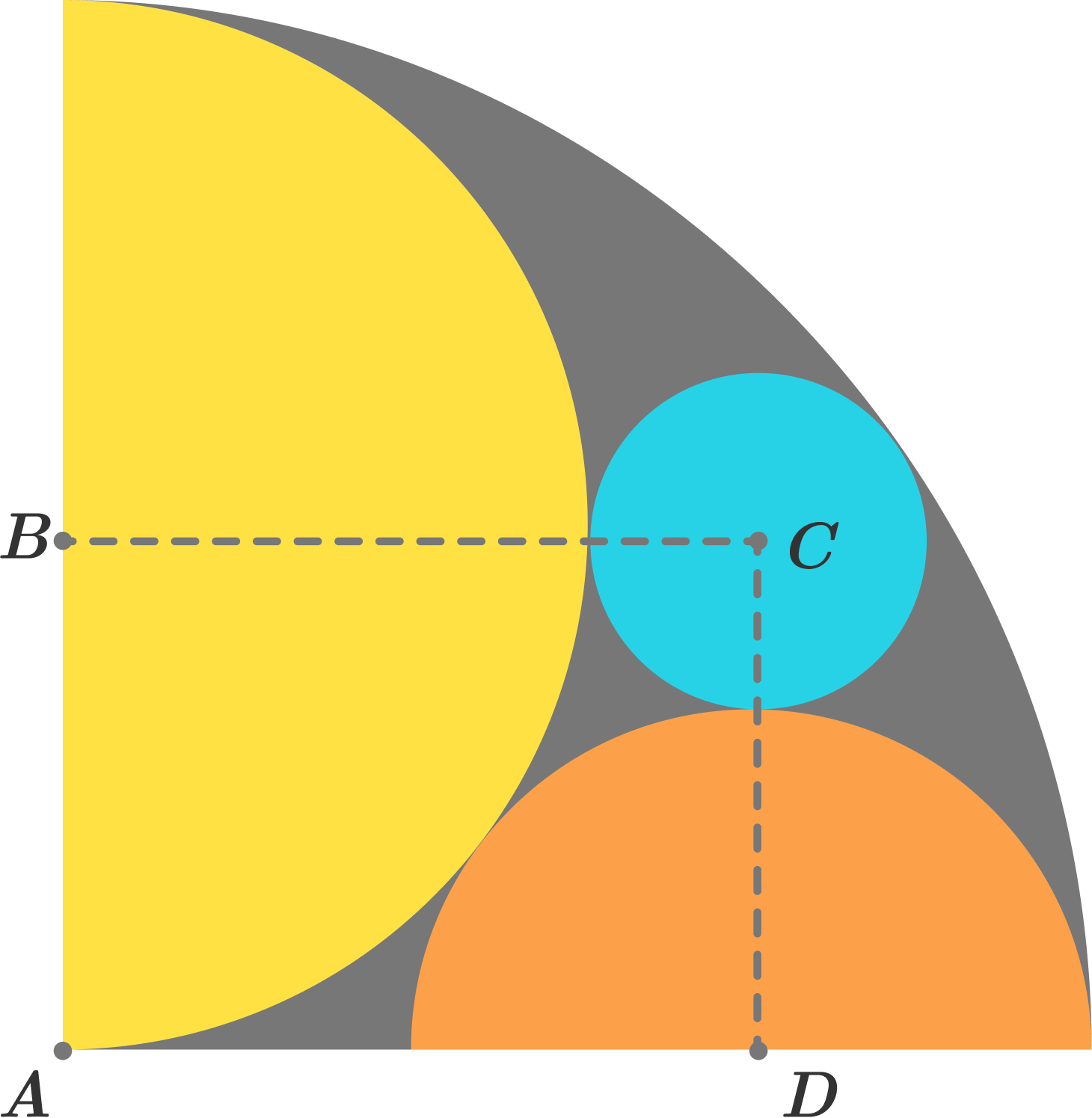A quarter-circle with radius $R$ is drawn. Inside it, two semicircles and a circle are drawn as shown in the above figure. If the area of the grey colored region is $\frac { \pi { R }^{ 2 } }{ X },$ find the value of $X.$